# Digital Resistor Sets Operating Power for Laser Driver

### 要約

Adding an op amp and a digital potentiometer to a standard laser-driver circuit produces a driver that varies the laser's power-set point in response to temperature, generating a photodiode current that is a linear function of the potentiometer value.

A similar version of this article appeared in the March 15, 2007 issue of ED magazine.

The MAX3740 VCSEL laser driver and DS1859 dual, temperature-controlled digital resistor are popular choices for SFP and SFF fiber-optic systems, due to their small size and high level of integration. The MAX3740 uses a monitoring photodiode and automatic power-control loop (APC) to compensate the laser power for temperature effects and aging. Photodiode responsivity can vary as much as 40%, so the system needs some additional compensation. That can be achieved with a digital resistor, which varies the power set-point in response to temperature.

A resistor between the MAX3740 reference pin (REF) and the power monitor photodiode (MD) sets the photodiode current. The power-control loop then drives the laser diode to the intensity that delivers that current. The problem with this approach is a too-low control voltage: the nominal voltage at MD is 1.6V and the nominal voltage at REF is 1.8V, leaving only 0.2V across the resistor for setting the photodiode current.

Digital resistors like those in the DS1859 can have minimum resistances as high as 1kΩ, which in turn gives a maximum current of only 200µA. The resulting current vs. resistance function is very nonlinear, with poor resolution at high currents. You can add a fixed resistor between REF and MD to raise the maximum current, but the adjustment range is still only 200µA. (Nor does the fixed resistor provide any improvement in nonlinearity and resolution.) A graph of photodiode current vs. DS1859 resistance (lower-left trace in Figure 1) shows the response of a circuit with series resistor value of 806Ω, which biases up the response by 248µA.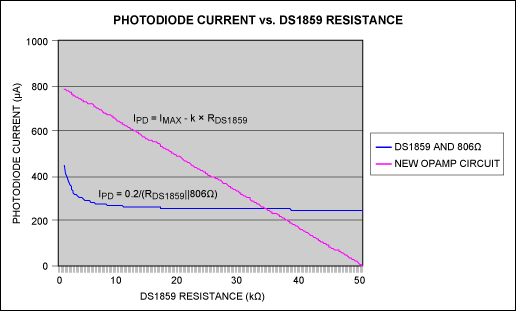Figure 1. The MAX3740 laser driver with external photodiode produces a nonlinear control voltage for the laser (lower-left trace). Adding a digital resistor and op amp (Figure 2) produces the linear control voltage shown. The solution to these problems (Figure 2) is to allow the resistor between REF and MD (R1) to set the maximum photodiode current, and then subtract a current proportional to the DS1859 resistance. The subtracted current comes from the op-amp output, which steals current from the photodiode through R2. The op amp shown was chosen for its small size (SC70 package) and low cost. It is powered by the same +3.3V power supply as the digital resistor (DS1859) and laser driver (MAX3740).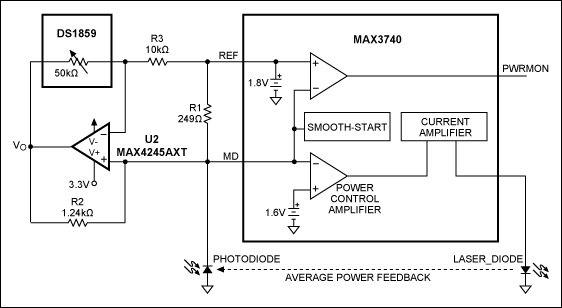Figure 2. An external op amp and digital resistor (DS1859) enable the MAX3740 laser driver to generate the linear control voltage shown in Figure 1.

The op amp generates a voltage (VO) proportional to the value of MD (REF - MD) and the DS1859 value. In turn, the voltage generates a current through R2 proportional to the difference between the voltages at VO and MD. The effects at MD cancel out, so the current through R2 depends only on (REF - MD), a stable 0.2V, and the DS1859 value. Current through the photodiode equals the current thru R1 (803µA) minus the current thru R2. Thus, photodiode current is a linear function of the potentiometer value, as illustrated in Figure 1. With appropriate resistor values, this circuit works with any value potentiometer and provides current over any range. Its only limitation is the current-drive capability of the op amp. Design calculations are:

Derivation of equations

Equation for op amp output voltageRearrange termsSubstitute 0.2V for reference voltage - modulation voltageEquation for current through R2 Substitute in equation for op amp output voltage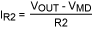Modultion voltage terms cancel out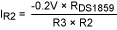Equation for current through R1 Substitute 0.2V for reference voltage - modulation voltage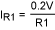Equation for current through photo diode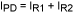Substitute equations for R1 current and R2 current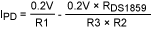Where
R1 = 249Ω
R2 = 1240Ω
R3 = 10000Ω
IPD = 787µA, DS1859 resistance = 1kΩ
IPD = -3.3µA, DS1859 resistance = 50kΩ

Design procedure

Select desired photodiode min and max currentDS1859 min and max valuesOp amp output voltage range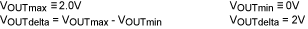Modulation voltage min and max